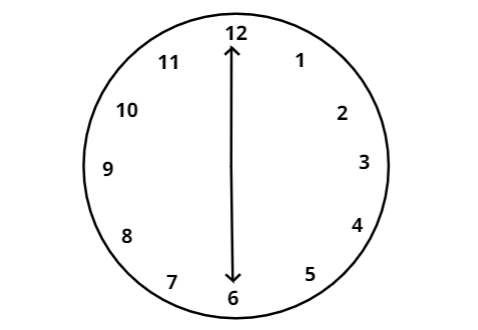QUESTION

# What is the degree of the angle at 6 o'clock?Since, there are 12 hours on the clock, each hour mark is$\dfrac{{360}}{{12}} = {30^0}$.
Therefore, when we calculate where the hour hand will be at 6 O'clock it is $(6)({30^0}) = {180^0}$
Hence, when time is 6 O'clock, angle is ${180^0}$. So it is a straight angle.